### A Demonstration of Using Characteristics on the Mixer Example from the Tutorial

Let's consider the main capabilities on a simple typical FlowVision example - namely, the mixer. The complete project is detailed in the Quick Start section of the FlowVision documentation.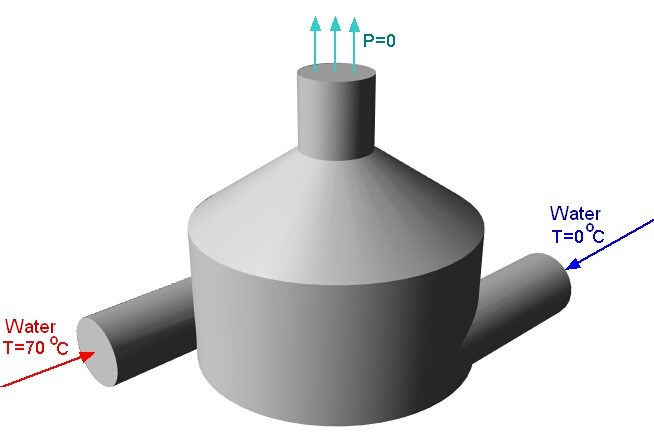In this task, there are two inlets: one for cold water, the other for hot, and the outlet is located at the top of the mixer. For the two inlets, we assign the boundary condition (BC) - normal mass velocity; and for the mixer outlet - zero total pressure. In order to be confident that the solution has converged and reached a stationary state, it is necessary to track the dynamics of changes in variables over time. If they do not change over time, then the calculation can be stopped. In the given task, it is reasonable to monitor the average velocity and temperature at the mixer outlet. It would also be useful to track the flow rate balance to make sure that our Phase does not “leak” anywhere, and that the amount of fluid introduced into the calculation domain is equal to the amount of fluid leaving it. We will accomplish all this using Characteristics.

We will not be applying a turbulence model. The selected grid is 20x20x20, the time step is 0.01 seconds.

To begin, you need to create three Supergroups: a Supergroup for “Hot Inlet”; a Supergroup for “Cold Inlet”; and a Supergroup for “Outlet”. We do this according to the corresponding Boundary Conditions.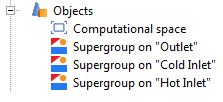Then, for each Supergroup, we create characteristics for the variables  which are of interest. For both of the Inlets let's create two characteristics - pressure, and speed. For the Supergroup on “Outlet”, create characteristics for pressure, speed, and temperature.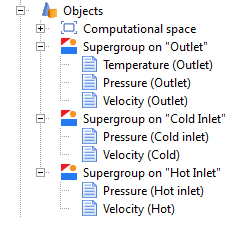Another application of Characteristics is to calculate secondary variables using formulas set by user variables. Let's look at an example of how to do this. Let’s say we want a Characteristic, which will contain the result of calculating the difference between the flow rate at the outlet of the mixer and the flow rates at the hot and cold inlet. To do this, first create a custom variable in the PrePostProcessor tab. Let's call this variable “Flow rate difference” and use a formula to set its value.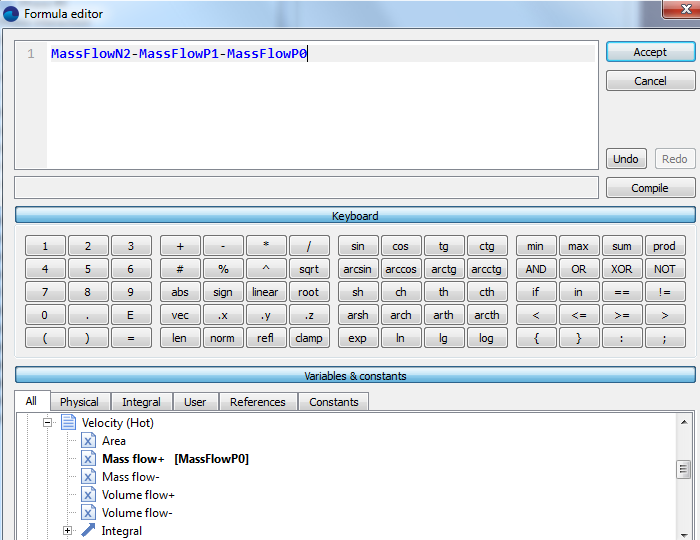Then it is necessary to create a Characteristic on the Supergroup object for “Outlet”. In its properties window in the Variable>Category field, select “User variables”, and in the Variable> Variable field, select our created variable “Flow rate difference”.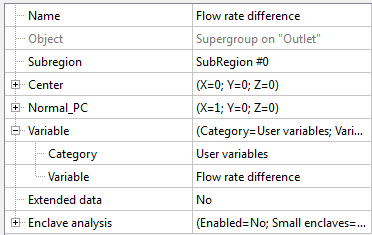Next, go to the Solver tab> Stop Conditions> User Values and create seven stop criteria. In the properties window of each Stop Criterion, set the corresponding object - the Characteristic based on which the calculations will be made.

To calculate the velocities at the selected BCs, select <f surf.> in the “variable” field. This will calculate the average value of the variable over the whole surface. Similarly, calculate the average outlet temperature. View the documentation for a more detailed description of additional features of FlowVision, as well as of the purpose of the other items in the variable field (such as <f mass +>, <f mass->, Standard deviation, Heat flux [W], etc).

We end up with the following stop criteria: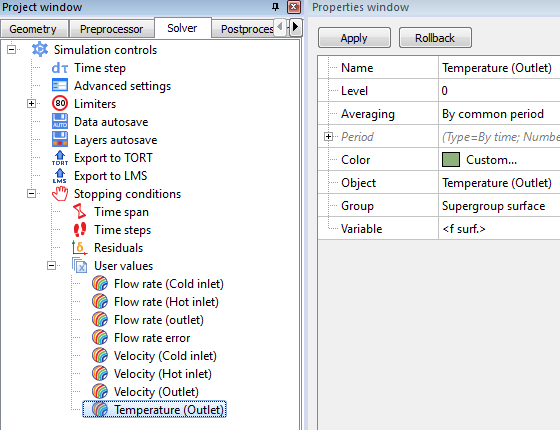This completes the preparation of the calculation. After starting the calculation, all of these values can be monitored in the Monitor Window.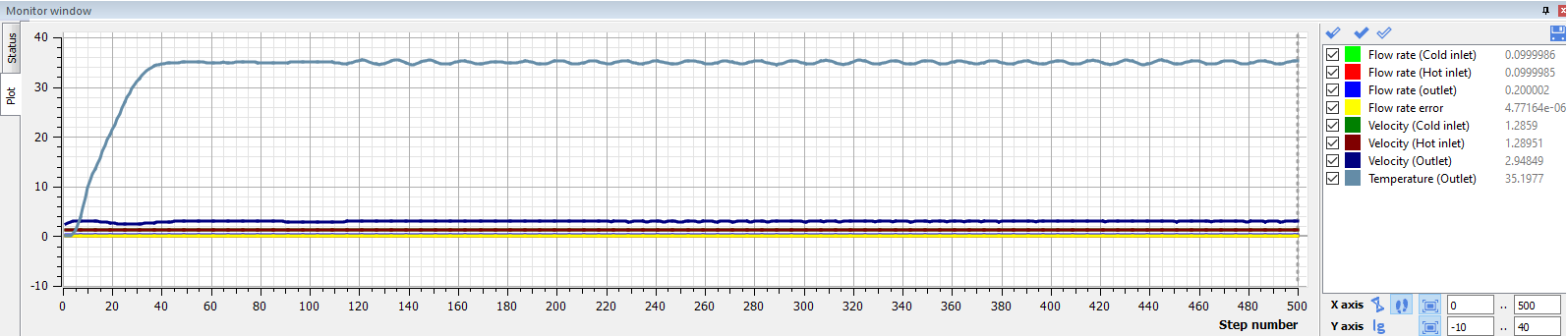You can select a variable of interest and monitor only its value alone. For example, consider the graph of the change of the average outlet temperature with time.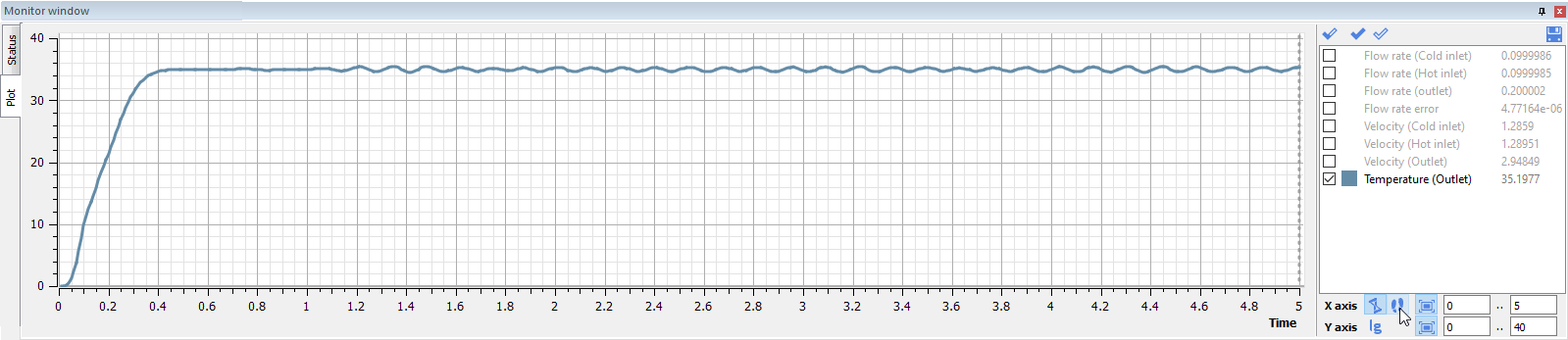Looking at this result, it can be seen that the problem has already reached a quasi-static solution at 1.2 seconds, and that the average outlet temperature is about 35 degrees.

However, the outlet velocity stabilized only at 2.4 seconds, and is approximately equal to 2.9 m / s: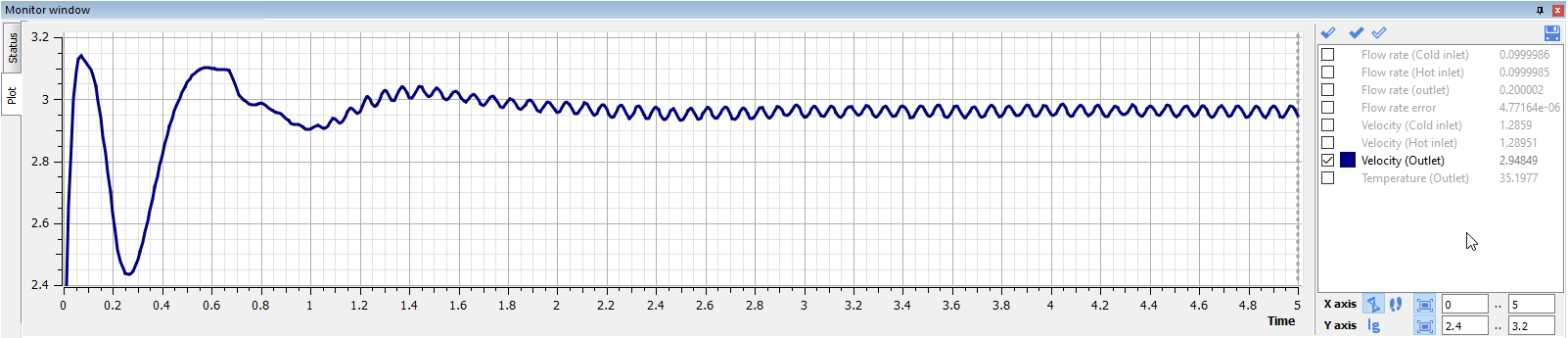The mass flow balance was slightly off zero initially, but at 0.6 seconds it has already leveled off and is fluctuating around zero: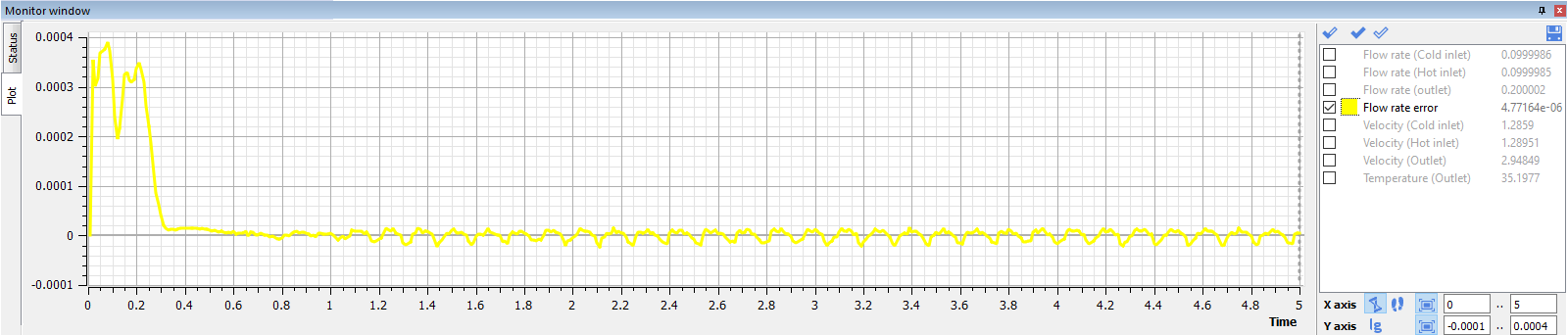The resulting graphs are unsteady. This is due to the fact that when preparing the model for calculation, it was chosen to use a large time step and a coarse mesh.

In this task, in order to achieve complete convergence, it is necessary to refine the mesh and decrease the time step. Then the values of the Characteristics will eventually assume constant values.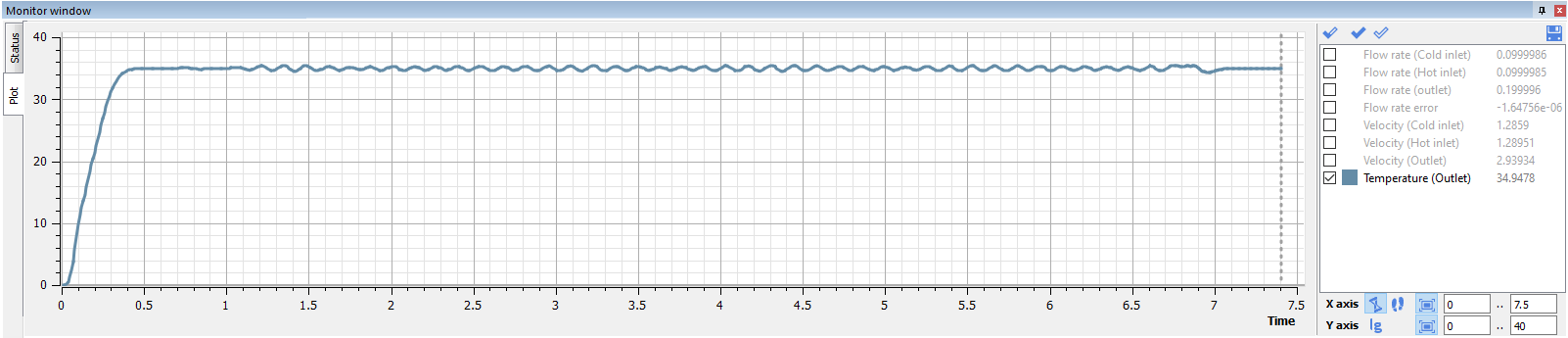Notice the resulting change in the average temperature over the outlet section after changes were applied (at 6.5 seconds the mesh was refined and the time step reduced).

The solution has completely stabilized, and at 7 seconds the average temperature has already settled on a constant value.